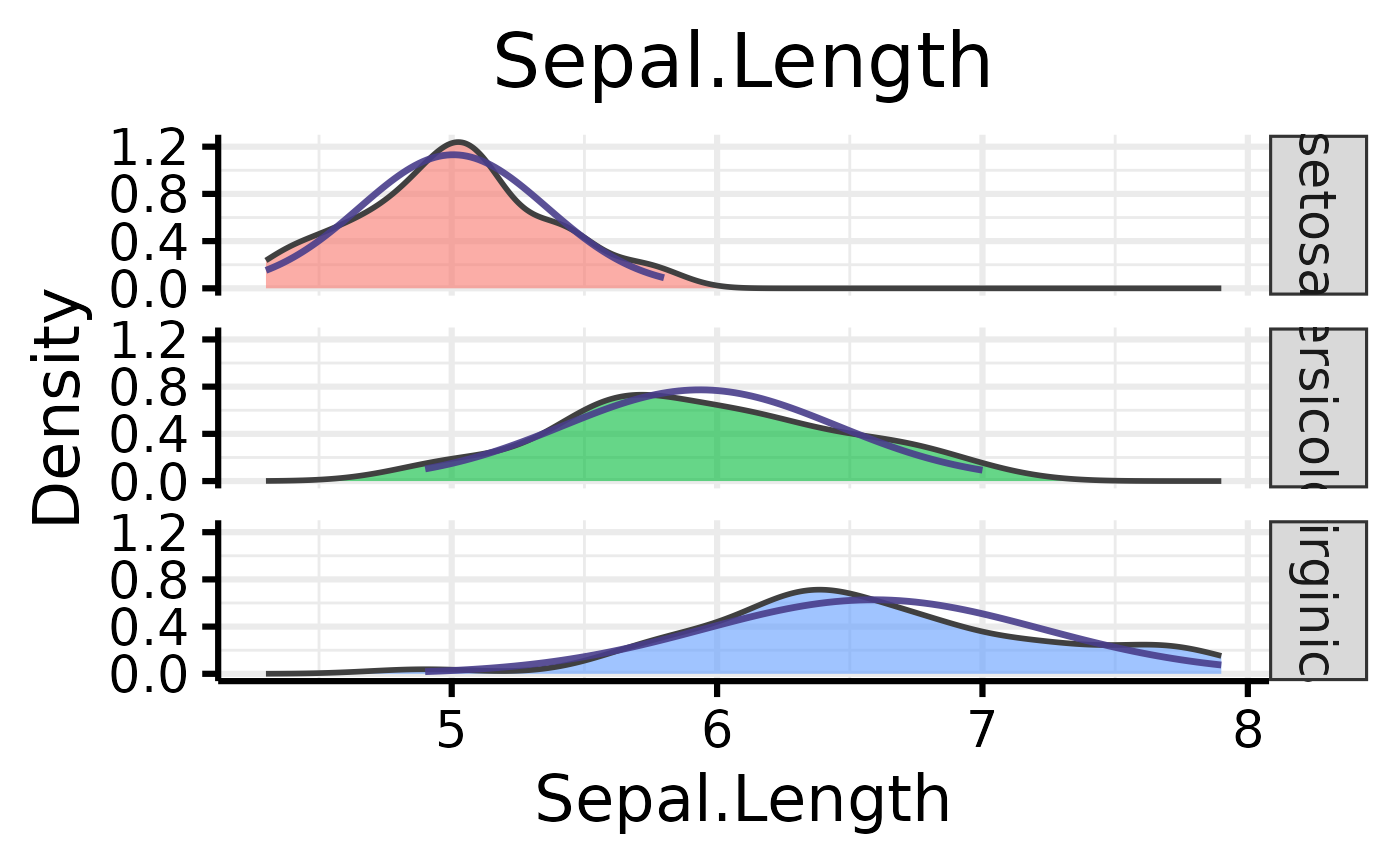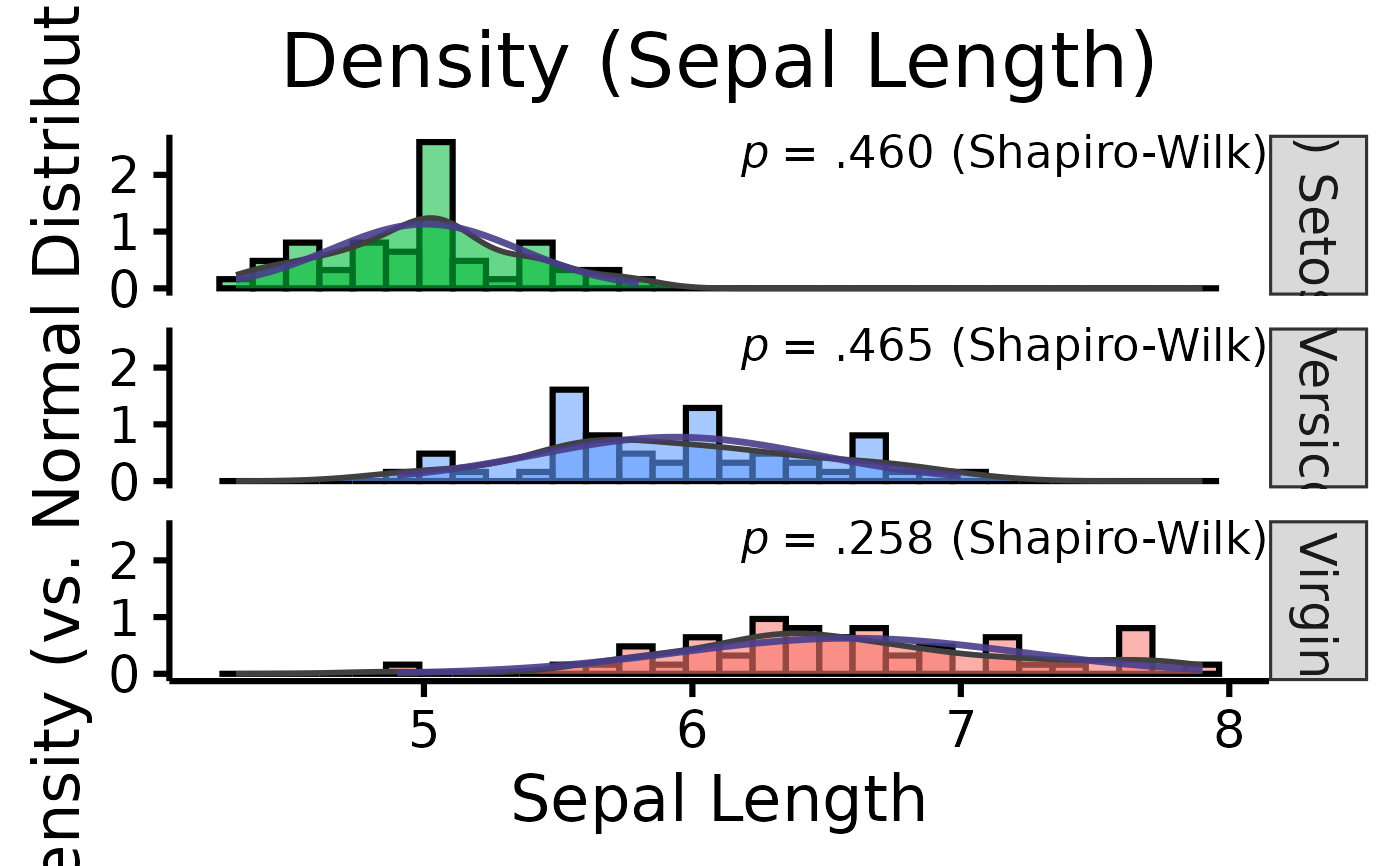Make nice density plots easily. Internally, uses na.rm = TRUE.

## Usage

nice_density(
data,
variable,
group,
colours,
ytitle = "Density",
xtitle = variable,
groups.labels = NULL,
grid = TRUE,
shapiro = FALSE,
title = variable,
histogram = FALSE,
breaks.auto = FALSE,
bins = 30
)

## Arguments

data

The data frame

variable

The dependent variable to be plotted.

group

The group by which to plot the variable.

colours

Desired colours for the plot, if desired.

ytitle

An optional y-axis label, if desired.

xtitle

An optional x-axis label, if desired.

groups.labels

The groups.labels (might rename to xlabels for consistency with other functions)

grid

Logical, whether to keep the default background grid or not. APA style suggests not using a grid in the background, though in this case some may find it useful to more easily estimate the slopes of the different groups.

shapiro

Logical, whether to include the p-value from the Shapiro-Wilk test on the plot.

title

The desired title of the plot. Can be put to NULL to remove.

histogram

Logical, whether to add an histogram

breaks.auto

If histogram = TRUE, then option to set bins/breaks automatically, mimicking the default behaviour of base R hist() (the Sturges method). Defaults to FALSE.

bins

If histogram = TRUE, then option to change the default bin (30).

## Value

A density plot of class ggplot, by group (if provided), along a reference line representing a matched normal distribution.

Other functions useful in assumption testing: nice_assumptions, nice_normality, nice_qq, nice_varplot, nice_var. Tutorial: https://rempsyc.remi-theriault.com/articles/assumptions

## Examples

# Make the basic plot
nice_density(
data = iris,
variable = "Sepal.Length",
group = "Species"
)# Further customization
nice_density(
data = iris,
variable = "Sepal.Length",
group = "Species",
colours = c("#00BA38", "#619CFF", "#F8766D"),
xtitle = "Sepal Length",
ytitle = "Density (vs. Normal Distribution)",
groups.labels = c(
"(a) Setosa",
"(b) Versicolor",
"(c) Virginica"
),
grid = FALSE,
shapiro = TRUE,
title = "Density (Sepal Length)",
histogram = TRUE
)